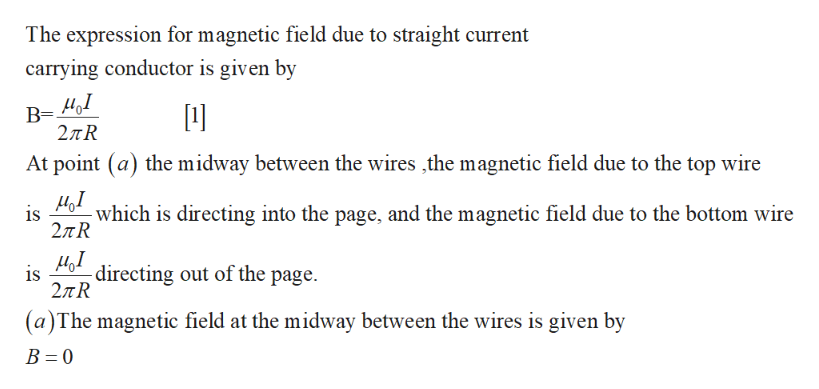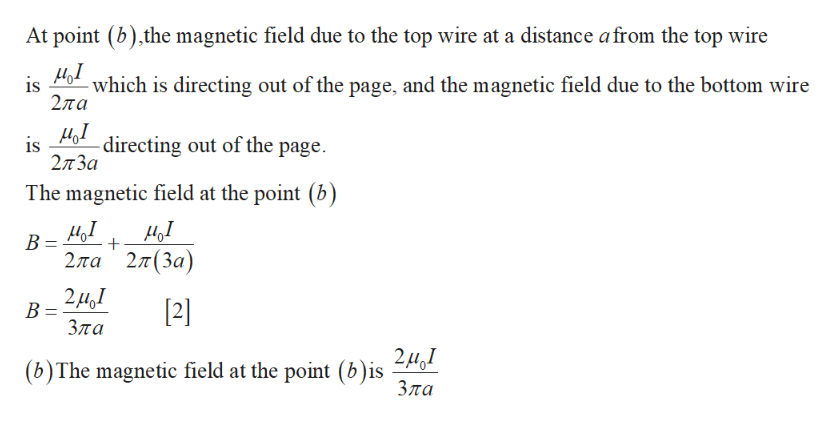# Two long, straight wires, one above the other, are seperated by a distance 2a and are parallel to the x−axis. Let the +y−axis be in the plane of the wires in the direction from the lower wire to the upper wire. Each wire carries current I in the +x−direction. Find B⃗  - the net magnetic field of the two wires at the following points in the plane of the wires.a) Midway between the wires. Express your answers in terms of the variables I, a, and magnetic constant μ0. b) At a distance a above the upper wire. Express your answers in terms of the variables I, a, and magnetic constant μ0.c) At a distance a below the lower wire. Express your answers in terms of the variables I, a, and magnetic constant μ0.

Question
12 views

Two long, straight wires, one above the other, are seperated by a distance 2a and are parallel to the x−axis. Let the +y−axis be in the plane of the wires in the direction from the lower wire to the upper wire. Each wire carries current I in the +x−direction. Find B⃗  - the net magnetic field of the two wires at the following points in the plane of the wires.

a) Midway between the wires. Express your answers in terms of the variables I, a, and magnetic constant μ0.

b) At a distance a above the upper wire. Express your answers in terms of the variables I, a, and magnetic constant μ0.

c) At a distance a below the lower wire. Express your answers in terms of the variables I, a, and magnetic constant μ0.

check_circle

Step 1

Write the expression for magnetic field due to straight current carrying conductor.help_outlineImage TranscriptioncloseThe expression for magnetic field due to straight current carrying conductor is given by В- 2лR At point (a) the midway between the wires the magnetic field due to the top wire М,1 which is directing into the page, and the magnetic field due to the bottom wire is 2лR is directing out of the page 27TR (a)The magnetic field at the midway between the wires is given by В - 0 fullscreen
Step 2help_outlineImage TranscriptioncloseAt point (b),the magnetic field due to the top wire at a distance afrom the top wire is which is directing out of the page, and the magnetic field due to the bottom wire 2ла is directing out of the page 2лЗа The magnetic field at the point (b) В- + 2ла 2л (За) 2д,1 В  Зла 2м.1 (b)The magnetic field at the point (b)is Зла fullscreen

### Want to see the full answer?

See Solution

#### Want to see this answer and more?

Solutions are written by subject experts who are available 24/7. Questions are typically answered within 1 hour.*

See Solution
*Response times may vary by subject and question.
Tagged in

### Physics